# Cone

A cone is a solid composed of a circle and its interior ( base ), a given point not on the plane of the circle ( vertex ) and all the segments from the point to the circle.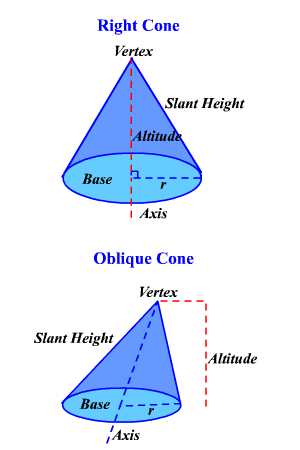The radius of the cone is the radius of the base. The altitude of the cone is the perpendicular segment from the vertex to the plane of the base. The height of the cone is the length of the altitude.

The axis of the cone is the segment whose endpoints are the vertex and the center of the base. If the axis is perpendicular to the plane of the circle, the cone is a right cone otherwise it is an oblique cone .

The slant height of a right cone is the length of the segment from the vertex of the cone to the circle of the base. Slant height is not defined for oblique cones.

A cone is closely related to a pyramid . So, the formulas for their surface areas and volume are related.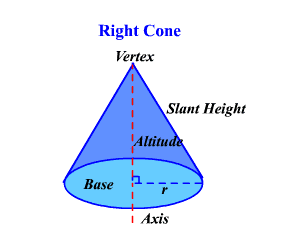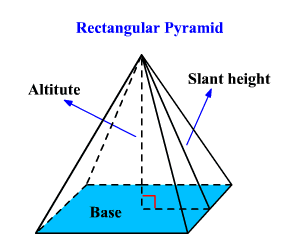Remember, the formulas for the lateral surface area of a pyramid is $\frac{1}{2}pl$ and the total surface area is $\frac{1}{2}pl+B$ .

Since the base of a cone is a circle, we substitute $2\pi r$ for $p$ and $\pi {r}^{2}$ for $B$ where $r$ is the radius of the base of the cone.

So, the formula for the lateral surface area of a right cone is L. S. A. = $\pi rl$ , where $l$ is the slant height of the cone.

Example 1:

Find the lateral surface area of a right cone if the radius is $4$ cm and the slant height is $5$ cm.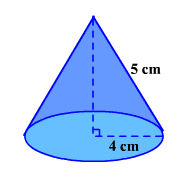The formula for the total surface area of a right cone is $\text{T}\text{.S}\text{.A}\text{.}=\pi rl+\pi {r}^{2}$ .

Example 2:

Find the total surface area of a right cone if the radius is $6$ inches and the slant height is $10$ inches.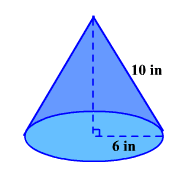Since slant height is undefined for an oblique cone, there are no formulas for the areas of oblique cones.

The volume of a circular cone is one-third the product of its altitude and the area of its base.  $\left(V=\frac{1}{3}Bh\right)$ .

Example 3:

Find the volume of a cone whose altitude is $15$ m and whose radius is $8$ m.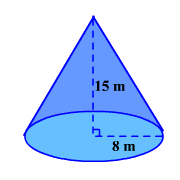$\begin{array}{l}V=\frac{1}{3}{\left(8\right)}^{2}\left(\pi \right)\left(15\right)\\ \text{\hspace{0.17em}}\text{\hspace{0.17em}}\text{\hspace{0.17em}}\text{\hspace{0.17em}}\text{\hspace{0.17em}}=320\pi \\ \text{\hspace{0.17em}}\text{\hspace{0.17em}}\text{\hspace{0.17em}}\text{\hspace{0.17em}}\text{\hspace{0.17em}}\approx 1005.31\end{array}$

Therefore, the volume of the cone is about .